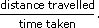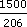Ratio, proportion and percentages

This free course is available to start right now. Review the full course description and key learning outcomes and create an account and enrol if you want a free statement of participation.

Free course

1.5 Speeds

Speed is the ratio of distance travelled to time taken. A runner's speed may be quoted in metres per second, miles per hour or kilometres per hour. The units are given as:

unit of distance per unit of time.

When you have a distance covered (such as a mile) and a time taken (such as four minutes) the average speed is defined as

•The formula for average speed applies even over a journey made up of several stages.

Example 6

In 1999, Hicham El Guerrouj held the record for running a mile. He covered the distance in just over 3 minutes 43 seconds. In the same year he also held the world record for the 1500 m race. He completed this distance in 3 minutes 26 seconds.

Work out:

• (a) his (average) speed in miles per hour for the 1 mile race;

• (b) his (average) speed in kilometres per hour for the 1500 metre race;

• (c) compare (a) and (b). In which race was his (average) speed faster assuming that 1.61 km = 1 mile?

• (a) To find the speed in miles per hour, you need the ratio of the distance in miles to time in hours. In 223 seconds (3 minutes 43 seconds) he ran 1 mile. Therefore in 1 second he would run 1/223 miles. In 3600 seconds (i.e. 1 hour), he would run 3600/223 miles i.e. 16.14 miles (to 2 d.p.). So the athlete's speed is 16.14 miles per hour (to 2 d.p.).

• (b) Hicham ran 1500 metres in 3 minutes 26 seconds (i.e. 206 seconds), so he ranmetres in 1 second. In 3600 seconds (1 hour) he runs 3600 ×metres. That is, 26 213.59 metres per hour. To convert to kilometres: divide by 1000 which gives 26.21 km per hour (to 2 d.p.).

• (c) To compare speeds in different units, you need to convert one to the other, say miles per hour to kilometres per hour. Since 1 mile is 1.61 km, 16.14 miles is 16.14 × 1.61 = 25.99 km (to 2 d.p.). Hence 16.14 miles per hour is the same as 25.99 km per hour. This is a lower speed than 26.21 km per hour. So Hicham ran the 1500 metre race faster, which is not surprising as it is a shorter distance.

MU120_4M3